10.5.2 Left-Looking Deletion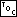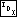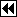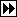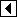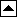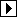The previous section implemented the “right-looking” form of deletion used elsewhere in libavl. Compared to deletion in a fully threaded binary tree, the benefits to using an RTBST with this kind of deletion are minimal:

• Cases 1 and 2 are similar code in both TBST and RTBST deletion.
• Case 3 in an RTBST avoids one tag copy required in TBST deletion.
• One subcase of case 4 in an RTBST avoids one tag assignment required in the same subcase of TBST deletion.

This is hardly worth it. We saved at most one assignment per call. We need something better if it's ever going to be worthwhile to use right-threaded trees.

Fortunately, there is a way that we can save a little more. This is by changing our right-looking deletion into left-looking deletion, by switching the use of left and right children in the algorithm. In a BST or TBST, this symmetrical change in the algorithm would have no effect, because the BST and TBST node structures are themselves symmetric. But in an asymmetric RTBST even a symmetric change can have a significant effect on an algorithm, as we'll see.

The cases for left-looking deletion are outlined in the same way as for right-looking deletion:

```388. <Step 2: Delete RTBST node, left-looking 388> =
if (p->rtbst_link == NULL)   {
if (p->rtbst_rtag == RTBST_CHILD)
{         <Case 1 in left-looking RTBST deletion 389>       }
else       {         <Case 2 in left-looking RTBST deletion 390>       }
} else   {
struct rtbst_node *r = p->rtbst_link;
if (r->rtbst_rtag == RTBST_THREAD)
{         <Case 3 in left-looking RTBST deletion 391>       }
else       {         <Case 4 in left-looking RTBST deletion 392>       }
}
```

This code is included in 380.

##### Case 1: p has a right child but no left child

If the node to delete p has a right child but no left child, we can just replace it by its right child. There is no right thread to update in p's left subtree because p has no left child, and there is no left thread to update because a right-threaded tree has no left threads.

The deletion looks like this if p's right child is designated x: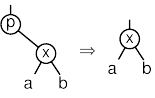```389. <Case 1 in left-looking RTBST deletion 389> =
```

This code is included in 388.

##### Case 2: p has a right thread and no left child

This case is analogous to case 2 in right-looking deletion covered earlier. The same discussion applies.

```390. <Case 2 in left-looking RTBST deletion 390> =
if (dir == 1)
```

This code is included in 388.

##### Case 3: p's left child has a right thread

If p has a left child r that itself has a right thread, then we replace p by r. Node r receives p's former right link, as shown here: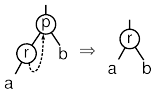There is no need to fiddle with threads. If r has a right thread then it gets replaced by p's right child or thread anyhow. Any right thread within r's left subtree either points within that subtree or to r. Finally, r's right subtree cannot cause problems.

```391. <Case 3 in left-looking RTBST deletion 391> =
r->rtbst_rtag = p->rtbst_rtag;
```

This code is included in 388.

##### Case 4: p's left child has a right child

The final case handles deletion of a node p with a left child r that in turn has a right child. The code here follows the same pattern as <Case 4 in TBST deletion 263> (see the discussion there for details). The first step is to find the predecessor s of node p:

```392. <Case 4 in left-looking RTBST deletion 392> =
struct rtbst_node *s;

for (;;)   {
if (s->rtbst_rtag == RTBST_THREAD)
break;

r = s;
}
```

This code is included in 388.

Next, we update r, handling two subcases depending on whether s has a left child:

```393. <Case 4 in left-looking RTBST deletion 392> +=
if (s->rtbst_link != NULL)
else   {
}
```

The final step is to copy p's fields into s, then set q's child pointer to point to s instead of p. There is no need to chase down any threads.

```394. <Case 4 in left-looking RTBST deletion 392> +=